Browse by Topic

# Centennial of Markov Chains

On January 23, 1913 of the Julian calendar, Andrey A. Markov presented for the Royal Academy of Sciences in St. Petersburg his analysis of Pushkin’s Eugene Onegin. He found that the sequence of consonants and vowels in the text could be well described as a random sequence, where the likely category of a letter depended only on the category of the previous or previous two letters.

At the time, the Russian Empire was using the Julian calendar. The 100th anniversary of the celebrated presentation is actually February 5, 2013, in the now used Gregorian calendar.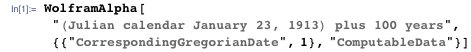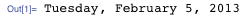To perform his analysis, Markov invented what are now known as “Markov chains,” which can be represented as probabilistic state diagrams where the transitions between states are labeled with the probabilities of their occurrences.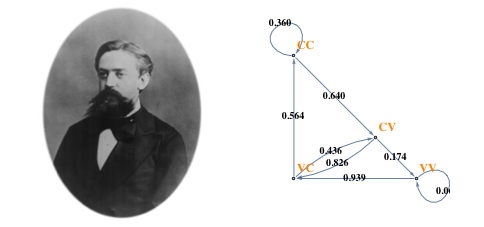### Alice in Wonderland: A Case Study

Here we repeat the analysis that Markov applied to Pushkin’s text on Alice’s Adventures in Wonderland, by Lewis Carroll. To this end, let’s define a function computing frequencies of elements of a list, returning results as rules.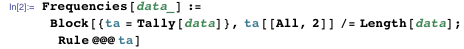First, extract words from the text, making them lowercase: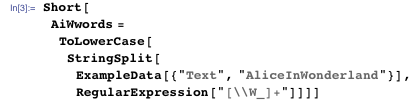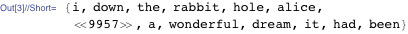Split the text into a sequence of letters: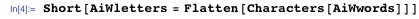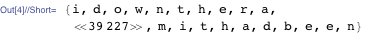Then classify them as vowels or consonants: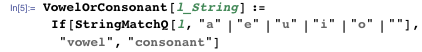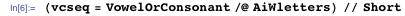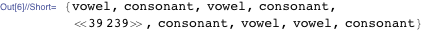And compute the frequencies of vowels and consonants in the text: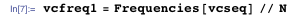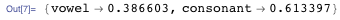Therefore, if we treat the text as a random sequence of either a vowel or a consonant, the probability of a vowel turning up is p = 0.3866.

Following Markov, let’s look at the frequencies of pairs of consecutive symbols: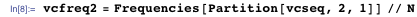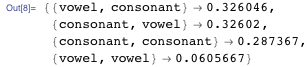Then we find the probabilities of a vowel or a consonant given by what precedes it: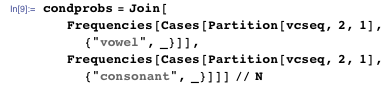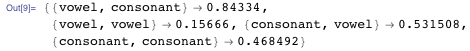In his paper, Markov observed that the sequence of vowels and consonants agreed much better with the model where the probability of a vowel depended on the preceding characters than with the model where it did not. Moreover, he found that the model where the probability depended on the two preceding characters agreed yet better.

Empowered with Mathematica, we can continue this investigation. Markov found that pairs of consecutive vowel-consonants carry more information than the sequence of vowel-consonants itself. One measure of the information stored in the data is the Entropy. The greater the entropy, the more information the data contains. Let’s compute it for the sequences of k-tuples of vowel-consonants (known as k-grams) for different values of k.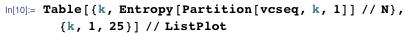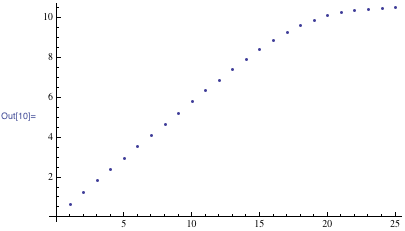The plot confirms Markov’s findings. Curiously, it also shows that 25-grams carry little more information that 20-grams.

The probabilistic 2-gram model describing the sequence is now known as a Markov chain process.

The Markov chain process describes the evolution of a probability distribution πn on a state space at step n. Assuming that the state space is finite (in this example it consists of two elements {“vowel”,”consonant”}), the probability distribution can be thought of as a vector, and the conditional transition probabilities can be arranged in a matrix P: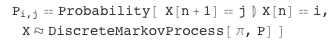In the case at hand, the transition matrix is: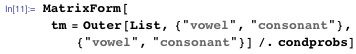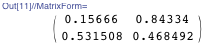Assuming the initial state is a vowel (encoded as 1), the 2-gram model is defined in Mathematica as follows: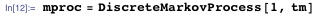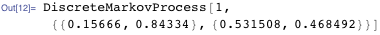With it, we can ask about the distribution of the distance between vowels in the text and compare the result with the data: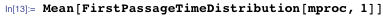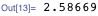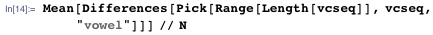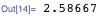Thus the Markov model accurately predicts that the average distance between vowels in the text is about 2.586 characters. The distributions of distances predicted by the model also agree well with those actually found in the text: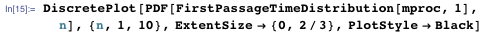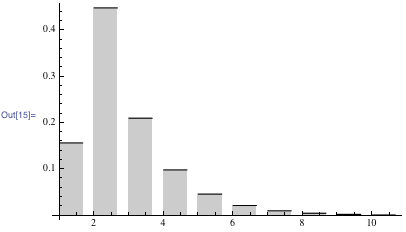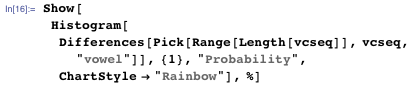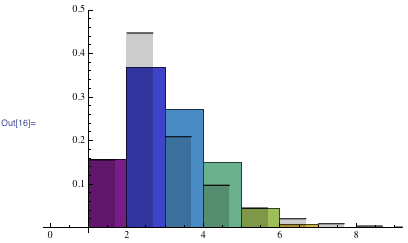### Text Generation

The text of Alice in Wonderland only uses 1,484 unique words: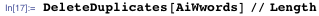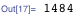Repeating the same analysis with words, rather than vowel-consonants, we find that 4-grams carry essential information: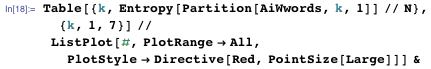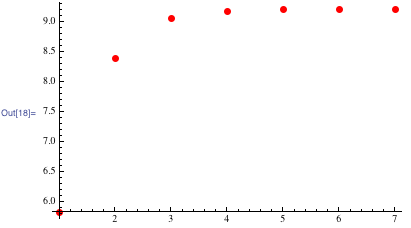With the 4-gram model, the frequency of {w1, w2, w3, w4} encodes the probability of w4, given the three most recent words w1 w2 w3.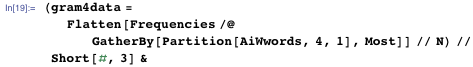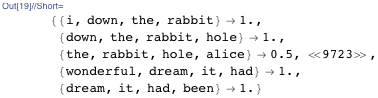We encode transitions {w1, w2, w3 } → {w2, w3, w4} in a directed graph, associating each edge with the “Probability” property, giving the conditional transition probability.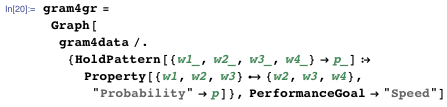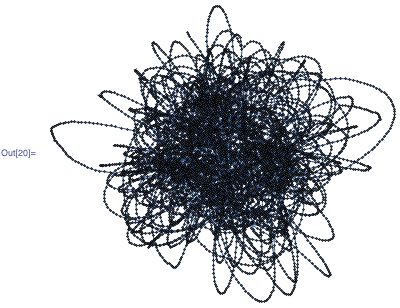Assuming the initial probability vector given by consecutive word pair frequencies defines the discrete Markov chain process: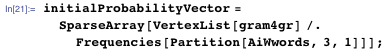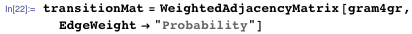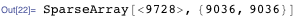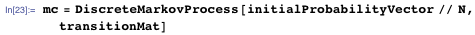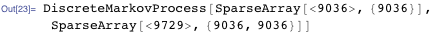Now we define a function, assembling a sequence of k-grams that resulted from walking the graph into a text.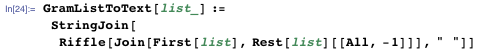We can now simulate the resulting Markov chain to generate a random 100-word text: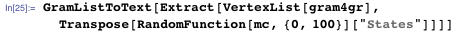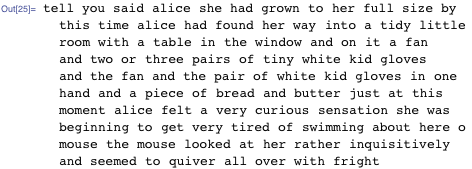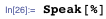### Linguistic Analysis

The graph associated with 4-grams has visibly long linear subgraphs, seen as long threads of vertexes. Words occurring along these threads will always appear in combination in the randomly generated text. It is interesting to examine length of such unchanged sequences.

These long subgraphs can be singled out by removing from the original graph all the vertexes that share more than two edges.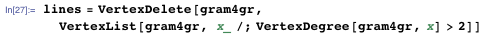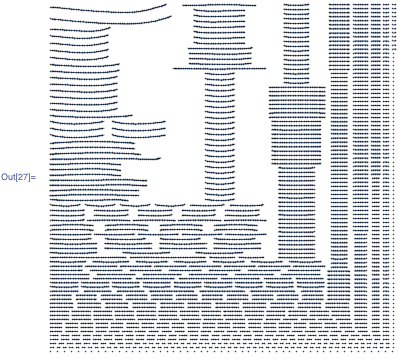We use WeaklyConnectedComponents to extract vertexes of these lines. After sorting them in the order in which they appeared in the text, we recreate the longest six such sequences. Each is a passage from the text (minus punctuation) in which every four-word sequence occurs uniquely in the text: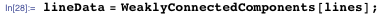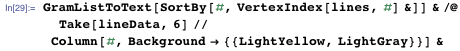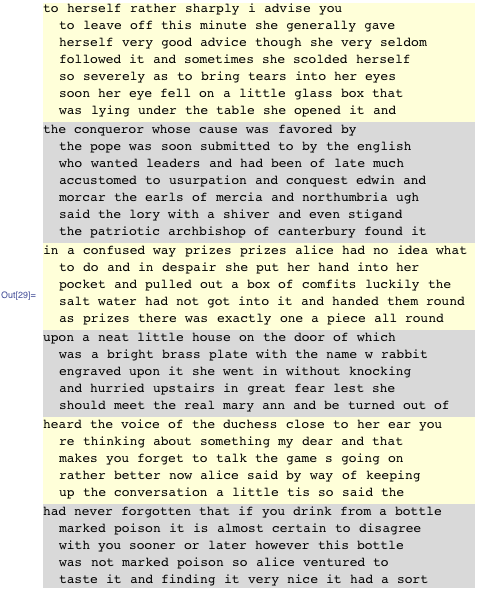As you can see, the six sequences are actually quite long. In fact, they are exceptionally long. A median length of such fragments is eight words. One could continue this analysis on other pieces of literature and compare the results, but we’ll leave that for another time.

Finite Markov processes in Mathematica can be used to solve a wide range of applied and theoretical problems. There are many examples at the Wolfram Demonstrations Project to help you celebrate 100 years of Markov chains.